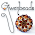20.7.12

Human Hypercube

Speaking of hypercubes, here's representation with people. We can build up to a hypercube by starting with a 0-dimensional point. 'Stretching' that in one direction gives us a 1-dimensional line segment. Stretch the line segment in a direction at 90° from the line segment and we get a 2-dimensional square. Stretching that 90° from the plane of the square gives us a 3-dimensional cubes. Now, stretch the cube at 90° from its volume (yes, I know it hurts to try to think like that. It's only impossible in the real-world, not in your mind!) and you'll have a 4-dimensional hypercube.

The pictures above show the changes from 0-d to 4-d.

Puzzles:
1. Describe how the number of vertices changes. Write a formula.
2. Describe how the number of line segments changes from one figure to the next. Write a formula.
3. Describe how the number of squares changes. Yep, formula.
4. Describe how the number of cubes changes.
5. Predict the properties of a 5-d hypercube. Can you draw one?

1 comment:

1.This is great. I like the way this representation of a hyper cube distinguishes the vertices and the edges in a human context. Nice.